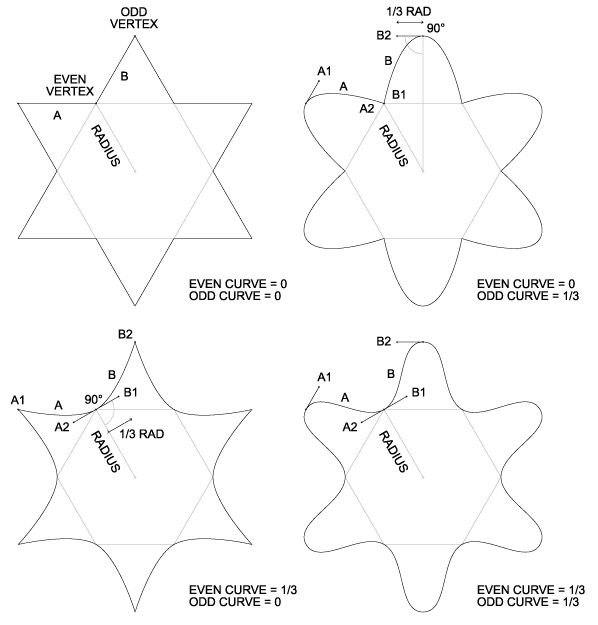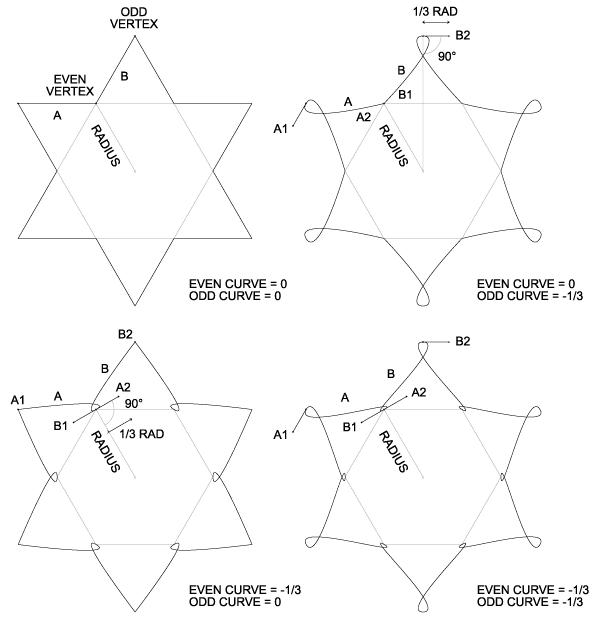### Even Curve

This is the curvature at even vertices, i.e. the vertices that are NOT affected by Star Factor and Pinwheel. The curvature at odd vertices is controlled separately, via the Odd Curve parameter. Curvatures are normalized such that positive values produce simple curves, negative values produce loops or curls, and at zero, there's no curvature. Note that curves require considerably more processing power than straight lines, and may reduce performance.

Each pair of adjacent vertices forms a Bťzier curve, with a control point at either end. The control points are constrained such that the vector between a control point and its corresponding vertex is always tangent. The vector's length is the ring's radius multiplied by the appropriate curvature (odd or even). Thus curvature doesn't vary with radius.

The following diagram shows the shapes that result from various combinations of odd and even positive curvature, with Star Factor = 1. An arbitrary curvature of 1/3 is used in all cases, to simplify the illustration.The following diagram shows the shapes that result from various combinations of odd and even negative curvature.